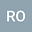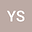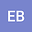$\psi$–Katugampola Fractional Derivatives and Integrals-Application to Mass–Spring Damper System
•••• Ramazan OZARSLAN,
• Erdal BAS
Ramazan OZARSLAN
Firat Universitesi
Author ProfileWe propose a new type of generalized fractional derivatives with respect to (wrt) another function. These new generalized fractional derivatives generalize $\psi$–Caputo, Riemann–Liouville (R–L) wrt another function, Caputo Hadamard wrt another function, R–L Hadamard wrt another function, Caputo, R–L, Caputo Hadamard and R–L Hadamard fractional derivatives. We propose a newly modified Laplace transform for linear $\psi$–Katugampola fractional differential equations (FDEs). Properties of this newly generalized Laplace transform are analyzed. Cauchy problems and mass-spring damper system with $\psi$–Katugampola fractional derivative are solved analytically by means of modified Laplace transform. Finally, a new numerical method is proposed for nonlinear $\psi$–Katugampola FDEs.